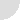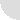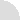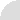LOGOLOG
a weblog of wordplay by Eric Harshbarger

## Slices of PI, Part 2

### Expectations

At the end of my first post about Slices of PI I spent a lot of time trying to impress the reader about the infinite length of PI's expansion. The random infinity of PI's digits allows for the expectation that any word, phrase, or body of text will eventually appear in PI (using the rules I defined), even though, so far, scanning the first 5 million digits, only a relatively few words have been found.

But the "expectation" is there.

What do I mean by that?

Well, in probabilistic terms it means what you might, um... well, what you might expect it to mean. An expectation of an event happening is how likely or often something would occur if the percentages of probability always came to be, exactly as calculated.

If you were to roll a standard, fair 6-sided die six times, the expectation that a "1" would be rolled would be 1.00 (or 100%). Since there is a 1 in 6 chance a roll would produce a 1 each time, and you are rolling the die six times, the expectation is 1/6 * 6 = 1.

Now, that doesn't mean you must roll a 1, or that you won't roll multiple 1s. It just means that you would expect to roll one 1.

In the Slices of PI project, what can we say about our probabilistic expectations? We've seen how quickly the occurance of generated words drop off, but let's look at it a bit more rigorously. How many digits of PI should we expect to use up hunting for these words?

Well, for a specific word of length L, the chance that that word will be generated from the modular arithmetic used on essentially random numbers is [1/26]^L (one twenty-sixth raised to the Lth power).

For a two letter word that means the chance that "DO" is generated for a random Slice Size is 1 in 676 (== 26 * 26). That's also the same chance of possibily generating the non-word, "VH"... it just happens to be the case that "DO" conveys meaning to us in our English intepreting brains.

That 1 in 676 chance was independent of the Slice Size. So, after 676 Slice Size choices, we would have an expectation of 1.00 that "DO" would be produced. Those 676 choices for Slice Sizes can most easily be chosen by incrementing Slice Size from 1 through 676. So, for a two letter word we would expect that in order to generate the word DO we would need 2 * 676 = 1352 digits of PI (two slices, the largest Slice Size for those slices being 676 each).

Now, by the same arguement, we would expect to generate any and all of the two letter words within that threshold of Slice Sizes. But, again, what we expect is not necessarily what we will get.

From the previous article we saw that we didn't find the last two letter word, MU, until S=4852 (using a total of twice that many digits, 9704).

If this seems contradictory, think again about the six die rolls mentioned above. Each digit, 1 through 6, would have an expectation of being rolled once in those six rolls (no digit would be more or less likely to come up than the others on a fair die). But if you actually do the experiment I doubt you actually will roll one of each number (disregarding order).

Oh, the mind-twisting joys of probability!

Back to our expectations of Slices of PI. You can probably see why, very quickly, the number of digits of PI we need just to fulfill our (likely unmet) expectations grows immensely. It's based on powers of 26...

1352 is not a lot of digits for a two letter word search, but consider how that number jumps when a specific five letter word is sought: 26 * 26 * 26 * 26 * 26 * 5 = 59,406,880 (the 5 multiple is there because we are looking to see the total number of digits of PI needed; each of the Slices would be 26^5 long, and there are 5 of them needed for a five letter word).

So, suddenly, if we want our expectation to reach 100%, and we are concerning ourselves with five letter words, we need over 59 million digits of PI.

Now, keep in mind, that there are a lot more five letter words than two letter words, so if we are just searching for any five letter word, not a specific one, that helps our chances of something valid being produced, but the word count is certainly not growing as quickly as the Slice Size requirements (and word count among growing word sizes soon levels off, then declines, anyway).

This is all to say that we would expect that all of the five letter words would be generated using the first, roughly 60 million digits of PI. But, again, it's quite likely that in reality we'd need a lot more than that.

Finding when the "complete works of Shakespeare" are embedded in Slices of PI might now seem a bit more daunting. The size of the numbers we're needing becomes mind-blowing. Even the simple title (not the whole poem), MARYHADALITTLELAMB, would require 18 * 26^18 digits to reach an expectation of 1.00. But, to the flipside again, even 500 septillion digits are easily grabbed from the infinity of PI, given enough time and resources.

PI never runs out...

Enough talk about expectations. Let's now examine the rules I defined for the Slices of PI project.

### Arbitrariness

One will remember from the previous article how I consciously made the decision to not use the beginning "3" in the digits of PI; I wanted to just consider all of the digits after the decimal point. This was definitely an arbitrary decision. It had to be made one way or the other, the decision was not going to inherently affect the project (the whole idea of Slices of PI could certainly be done with the 3 included; the answers would be different, yes, but no less likely).

This was definitely not the only arbitrary rule defined. In fact, pretty much all of the rules I established for the Slices of PI project were just as arbitrary:

• the fact that the Slices are all disjoint (non-overlapping),
• all of the Slices are of equal size,
• how I assigned index values to the letters.
Any of these parameters could be altered. For example, instead of A=0, B=1, ..., Z=25, I could have defined Z=0, Y=1, ..., A=25. For that matter, why keep things in order? One could choose any assignment of the numbers 0-25 to the letters A-Z. In this case, the order of the words discovered would be changed, but the expectation of discovery would essentially remain the same.

One way we could have changed our expectations (and made the words "easier" to find) would be not requiring all of the Slice Sizes to be the same size. Just study strings of digits as they come up in PI's expansion, but not all the same size. For example, apply modular arithmetic to these numbers:

```1415 9 265 35 89 7 93238 4 6 26 43 383 2 79
```

Plenty of other things could be changed, too. Maybe we don't start at the beginning of the expansion each time... just look anywhere in the digits.

So many choices. I had to decide on something. When I defined the rules for Slices of PI, I wanted to choose parameters that seemed somewhat "in the middle" of difficulty. Rules that would require a computer to do analysis, yet would produce some results fairly quickly.

Still, the vast majority of results (words) would be out of reach of the casual analysis (even with a computer). I wanted there to be plenty of "mystery" left... if the parameters had been set to easy, and all of the words discovered quickly... where's the fun in that?

But, of course, the reader is welcome to define her own parameters and analyze PI however she sees fit.

Even at the most generous "settings", I think it will be a while before all of those Shakespearean plays are found.

If you cook up a way to find "Mary had a little lamb," however, do let me know.

-- Eric

P.S.: A couple of hours and a few emails after I finished this LOGOLOG entry, Mike Keith delivered something to me. Using the "variable slice size" alternative I described above (and with A=0, B=1,...), he produced a sequence of numbers:

```27 37 4 50 38 1 3 12 75 30...
```
(it goes on for MUCH longer: 129,529 numbers separated by spaces)

Each number in the sequence accounts for a string of that many digits of PI, starting at .1415926...

So, the 27 means use the first 27 digits of the expansion; then use the next 37 digits, then the next 4, and so on. By converting them mod 26 to the letter indexing scheme, one gets: THETRADGEDY...

This is a very abbreviated account of Mike's analysis. What he, in fact, produced was a complete encoding of the full text of Shakespeare's HAMLET!

He did this using the first 3,359,924 digits of PI.

So finding all of The Bard's texts within PI using this scheme might be quite doable, after all!

[14 January 2006]Archive```Your Name:

Your comment (HTML tags will be removed):

Day of week today is:

```